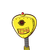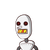# A triangular garden is equilateral in shape.The length of its side is 18 m. If the garden is fenced on all sides at the tota

A triangular garden is equilateral in shape.
The length of its side is 18 m. If the garden is fenced on all sides at the total cost of Rs. 2052, find the rate of fencing.

### 2 thoughts on “A triangular garden is equilateral in shape.<br />The length of its side is 18 m. If the garden is fenced on all sides at the tota”

1.the rate is rs. 38 per metre

Step-by-step explanation:

refer to the attachment

2.38 Rs/m

Step-by-step explanation:

Length of the triangles side = 18m

Cost of fencing = Rs.2052

Perimeter of triangle = 54

We have to calculate the rate is Rs/m. So we will find the answer in this way:

$$\frac{Rs.}{m}$$ = $$\frac{2052}{54}$$ =  38 Rs/m

Therefore, the rate of fencing is 38 Rupees per meter.

___________________________

Hope it helps